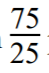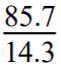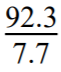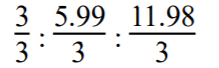# Carbon combines with hydrogen to form three binary compounds X, Y and Z. The percentage of hydrogen in X, Y and Z is 25%, 14.3% and 7.7% respectively, which law of chemical combination is illustrated by this data?

Carbon combines with hydrogen to form three binary compounds X, Y and Z. The percentage of hydrogen in X, Y and Z is 25%, 14.3% and 7.7% respectively, which law of chemical combination is illustrated by this data?

703

Solution:
Given,
In the compound X,
% of hydrogen = 25% & % of carbon = (100 – 25)% = 75% 25 parts by weight of hydrogen combine with 75 parts by weight of carbon.
1 part by weight of hydrogen combine withparts by weight of carbon
= 3 parts by weight of carbon

In the compound Y,
% of hydrogen = 14.3% & % of carbon = (100 – 14.3)% = 85.7% 14.3 parts by weight of hydrogen combine with 85.7 parts by weight of carbon
1 part by weight of hydrogen combine withparts by weight of carbon
=5.99 parts by weight of carbon

In the compound Z,
% of hydrogen = 7.7% & % of carbon = (100 – 7.7)% = 92.3% 7.7 parts by weight of hydrogen combine with 92.3 parts by weight of carbon
1 part by weight of hydrogen combine withparts by weight of carbon
= 11.98 parts by weight of carbon Hence the ratio of weight of carbon which combines with constant weight of hydrogen i.e. 1 part by weight of hydrogen is 3 : 5.99 : 11.98 == 1 : 1.99 : 3.99 i.e. 1 : 2 : 4 which is simple whole number ratio. Hence this data illustrate law of multiple proportions.